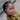# Understanding increment / decrement PREFIX or POSTPREFIX operator

Key:

Increment or Decrement operator can appear in two forms, the POSTPREFIX `x++ or x--` and PREFIX `++x or --x` and it can be quite confusing.

Understanding how both works will enable you know how to apply them in your project.

POSTPREFIX

This returns the value of its operand BEFORE adding 1 to it - Increment

This returns the value of its operand BEFORE subtracting 1 from it - Decrement

``````// increment

let x = 2

const r1 = x++ = 2 //it returned the valued the value before adding 1, meanwhile x is 3

const r2 = x++ + 2 = 5 ``````
``````// decrement

let x = 5

const r3 = x-- = 5 //it returned the value before subtracting 1, x= 4

const r4 = x-- + 2 = 6``````

PREFIX

This returns the value of its operand AFTER adding 1 to it - Increment

This returns the value of its operand AFTER subtracting 1 from it - Decrement

``````let x = 5

const r5 = ++x = 6 //it returned the value after adding 1

const r6 = ++x + 2 = 9``````
``````let x = 5

const r7 = --x = 4 //it returned  the value after subtracting 1

const r8 = --x + 2 = 5``````

Let’s mix both and see how it works

``````let x = 1

const a = x++ + x++= 3

const b = ++x + ++x = 9

const c = x++ + ++x = 12

const d = ++x + x++ = 16``````

try it out on your favorite code editor or you can view it on codepen
https://codepen.io/ojudith/pen/MWwZQxB

#programmingArticles written with 💛 by
Judith Oiku